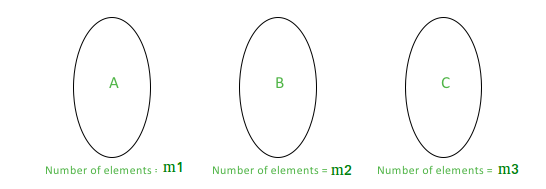# Number of Triangles that can be formed given a set of lines in Euclidean Plane

Given a set L = {l1, l2, ………, ln} of ‘n’ distinct lines on the Euclidean Plane. The ith line is given by an equation in the form aix + biy = ci. Find the number of triangles that can be formed using the lines in the set L. Note that no two pairs of lines intersect at the same point.

Note: This problem doesn’t mention that the lines can’t be parallel which makes the problem difficult to solve.

Examples:

```Input: a[] = {1, 2, 3, 4}
b[] = {2, 4, 5, 5}
c[] = {5, 7, 8, 6}
Output: 2
The number of triangles that can be formed are: 2

Input: a[] = {1, 2, 3, 2, 4, 1, 2, 3, 4, 5}
b[] = {2, 4, 6, 3, 6, 5, 10, 15, 20, 25}
c[] = {3, 5, 11, 10, 9, 17, 13, 11, 7, 3}
Output: 30
The number of triangles that can be formed are: 30
```

### Naive Algorithm

The naive algorithm can be described as:

1. Pick up 3 arbitrary lines from the set L.
2. Now check if a triangle can be formed using the selected 3 lines. This can be done easily by checking that none of them is pairwise parallel.
3. Increment the counter if the triangle can be formed.

Time Complexity: There are nC3 triplets of lines. For each triplet, we have to do 3 comparisons to check that any 2 lines are not parallel which means the check can be done in O(1) time. This makes the naive algorithm O(n3).

### Efficient Algorithm

This can also be achieved in O(n log n). The logic behind the efficient algorithm is described below.

We divide the set L in various subsets. The formation of subsets is based on slopes i.e. all the lines in a particular subset have the same slope i.e. they are parallel to each other.

Let us consider three sets (say A, B and C). For a particular set (say A) the lines belonging to this are all parallel to each other. If we have A, B and C, we can pick one line from each set to get a triangle because none of these lines will be parallel. By making the subsets we have ensured that no two lines which are parallel are being picked together.Now if we have only these 3 subsets,

```Number of triangles = (Number of ways to pick a line from A) *
(Number of ways to pick a line from B) *
(Number of ways to pick a line from C)
= m1*m2*m3
Here m1 is count of elements with first slope (in Set A)
Here m2 is count of elements with first slope (in Set B)
Here m3 is count of elements with first slope (in Set C)
```

Similarly, if we have 4 subsets, we can extend this logic to get,
Number of triangles = m1*m2*m3 + m1*m2*m4 + m1*m3*m4 + m2*m3*m4

For number of subsets greater than 3, If we have ‘k’ subsets, our task is to find the sum of number of elements of the subset taken 3 at a time. This can be done by maintaining a count array. We make a count array where counti denotes the count of the ith subset of parallel lines.

```We one by one compute following values.
sum1 = m1 + m2 + m3 .....
sum2 = m1*m2 + m1*m3 + ... + m2*m3 + m2*m4 + ...
sum3 = m1*m2*m3 + m1*m2*m4 + ...... m2*m3*m4 + ....
```

 `// C++ program to find the number of ` `// triangles that can be formed ` `// using a set of lines in Euclidean ` `// Plane ` `#include ` `using` `namespace` `std; ` ` `  `#define EPSILON numeric_limits::epsilon() ` ` `  `// double variables can't be checked precisely ` `// using '==' this function returns true if ` `// the double variables are equal ` `bool` `compareDoubles(``double` `A, ``double` `B) ` `{ ` `    ``double` `diff = A-B; ` `    ``return` `(diff

Output:

```The number of triangles that can be formed are: 2
```

Time Complexity: All the loops in the code are O(n). The time complexity in this implementation is thus driven by the sort function used to sort the slope array. This makes the algorithm O(nlogn).

This article is contributed by Aanya Jindal. If you like GeeksforGeeks and would like to contribute, you can also write an article using contribute.geeksforgeeks.org or mail your article to contribute@geeksforgeeks.org. See your article appearing on the GeeksforGeeks main page and help other Geeks.